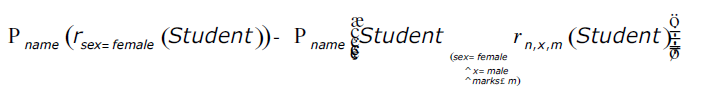# GATE | GATE-CS-2004 | Question 51

Consider the relation Student (name, sex, marks), where the primary key is shown underlined, pertaining to students in a class that has at least one boy and one girl. What does the following relational algebra expression produce? (Note: r is the rename operator).The condition in join is “(sex = female ^ x = male ^ marks ≤ m)”
(A) names of girl students with the highest marks
(B) names of girl students with more marks than some boy student
(C) names of girl students with marks not less than some boy students4)
(D) names of girl students with more marks than all the boy students

Explanation:

```The above relational algebra expression has two sub expressions.
The first one takes as input the Student relation (Student) and filters
out all the tuples where sex=female(r sex=female (Student))
and then projects their names (P name r sex=female (Student)).
So we get a new relation with names of all the female students.
```
```The second one takes as input the Student relation and performs a rename
operation on one with attributes name, sex and marks renamed as n, x, m
respectively (r n, x, m(Student)) and then followed by a self-Cartesian
product on the Student relation. The condition (sex = female ^ m = male ^ marks ≤ m)
filters tuples with all female students from the first relation,
male students from the second relation and performs a Cartesian product where
marks of the female student is either less than or equal to a male student and
then projects their names. So we get a new relation with names of all female
students whose marks are lesser than at least one of the male student.
```

The difference operator(-) between the two subexpressions gives the names of all
female students whose marks are more than all male students of the class.
(From all the female students’ names we remove all those whose marks are at
least more the one male student)

This explanation has been contributed by Yashika Arora.

Quiz of this Question

My Personal Notes arrow_drop_up
Article Tags :

Be the First to upvote.

Please write to us at contribute@geeksforgeeks.org to report any issue with the above content.